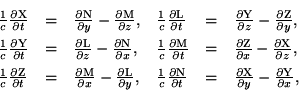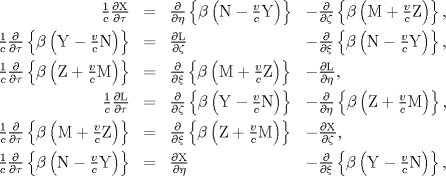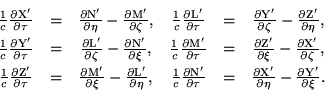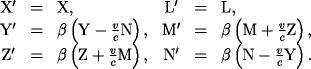# Electric Field Strength Comservation in Direction of Motion

(Sorry for the typo in the title. I don't know how to get rid of it)

Recently I was looking at the Lorentz co-variance of F=dA (the Faraday 2-form) and *F=*dA (the Maxwell 2-form). I realized that Lorentz co-variance implies that the electric field strength and magnetic field strength are invariant in the direction of motion. In Einstein's notation in Zur Elektrodynamik bewegter Körper (Engl trans.:ON THE ELECTRODYNAMICS OF MOVING BODIES), X=X' and L=L'. It struck me as interesting that he had to assume the invariance in order to prove the co-variance of Maxwell's equations:

Maxwell's eqns:transformed:and now in the new variables:Notice how he assumes X=X' and L=L'. This was no small assumption since it was at the time something Poincare was wanting to prove. The proof is, it seems, because it is necessary to establish co-variance, an established fact by then - otherwise you could measure the absolute velocity of the Earth through the luminiferous aether.

Further along he states explicitly:vanhees71
Gold Member
It's simply the transformation law for a 2nd-rank tensor,
$$F'^{\mu \nu}={\Lambda^{\mu}}_{\rho} {\Lambda^{\nu}}_{\sigma} F^{\rho \sigma}.$$
Plugging in the components in 1+3-dimensional notation, i.e., for $\mu \in \{1,2,3 \}$
$$F^{0 \mu}=\partial^{0} A^{\mu}-\partial^{\mu} A^0=\partial_0 A^{\mu} + \partial_{\mu} A^0=\dot{A}^{\mu}+\partial_{\mu} \Phi=-E^{\mu},$$
and for $\mu,\nu \in \{1,2,3 \}$
$$F^{\mu \nu}=\partial^{\mu} A^{\nu} - \partial^{\nu} A^{\mu}=-\partial_{\mu} A^{\nu} + \partial_{\nu} A^{\mu}=\epsilon^{\nu \mu \rho} B^{\rho},$$
you find the transformation law in terms of $(\vec{E},\vec{B})$ for a boost,
$$\begin{split} \vec{E}'&=\gamma(\vec{E}+\vec{\beta} \times \vec{B})-\frac{\gamma^2}{1+\gamma} \vec{\beta}(\vec{\beta} \cdot \vec{E}),\\ \vec{B}'&=\gamma(\vec{B}-\vec{\beta} \times \vec{E})-\frac{\gamma^2}{1+\gamma} \vec{\beta}(\vec{\beta} \cdot \vec{B}), \end{split}$$
where $\vec{\beta}=\vec{v}/c$ and $\gamma=1/\sqrt{1-\vec{\beta}^2}$. As you see, the components in boost direction are indeed unchanged.

Projecting to the parts in direction of $\vec{\beta}$ and perpendicular to it gives
$$\begin{split} \vec{E}_{\parallel}'&=\vec{E}_{\parallel},\\ \vec{B}_{\parallel}'&=\vec{B}_{\parallel},\\ \vec{E}_{\perp}'&=\gamma(\vec{E}_{\perp}+\vec{\beta} \times \vec{B}),\\ \vec{B}_{\perp}'&=\gamma(\vec{B}_{\perp}-\vec{\beta} \times \vec{E}). \end{split}$$

•1 person